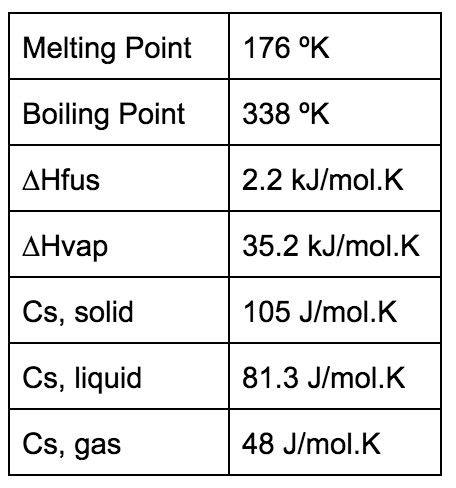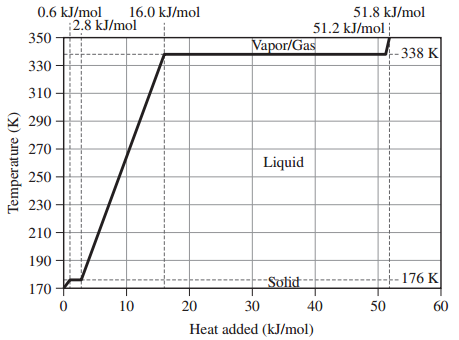# Problem: Assume that the values given here for 1 mole of methanol are constant over the relevant temperature ranges.Given the heating curve for 1 mole of methanol beginning at 170 K and ending at 350 K answer the following questions:What is the value of q gas for methanol?

🤓 Based on our data, we think this question is relevant for Professor Wolfman's class at BC.

###### FREE Expert Solution

q gas → heat absorbed in gas phase.

Given:

n = 1 mol

cgas = 48 J/mol-K

Ti = 338 K

Tf = 350 K

Calculate qgas:###### Problem Details

Assume that the values given here for 1 mole of methanol are constant over the relevant temperature ranges.Given the heating curve for 1 mole of methanol beginning at 170 K and ending at 350 K answer the following questions:What is the value of q gas for methanol?